# Complex projective space has orientation-reversing self-homeomorphism iff it has odd complex dimension

## Statement

Consider a complex projective space$\mathbb{P}^n(\mathbb{C})$, also denoted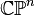$\mathbb{C}\mathbb{P}^n$, for$n$ a natural number. This has complex dimension$n$ (as a complex manifold) and real dimension$2n$ (as a real manifold). Moreover, for all values of$n$, it is a compact connected orientable manifold.

The claim is that:

• For$n$ odd, i.e.,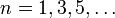$n = 1,3,5,\dots$ (so real dimensions$2,6,10,\dots$),$\mathbb{C}\mathbb{P}^n$ possesses an orientation-reversing self-homeomorphism. Viewed on homology, this is a homeomorphism that sends the fundamental class to its negative.
• For$n$ even, i.e.,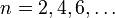$n = 2,4,6,\dots$ (so real dimensions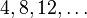$4,8,12,\dots$),$\mathbb{C}\mathbb{P}^n$ does not possess an orientation-reversing self-homeomorphism. Viewed on homology, there is no homeomorphism that sends the fundamental class to its inegative.

## Facts used

1. Cohomology of complex projective space

## Proof

The proof relies on the cohomology ring structure of complex projective space. The key idea is that if we take a generator for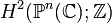$H^2(\mathbb{P}^n(\mathbb{C});\mathbb{Z})$, the$n^{th}$ power of this under the cup product gives a fundamental class.

Any self-homeomorphism of$\mathbb{P}^n(\mathbb{C})$ must send a generator of$H^2(\mathbb{P}^n(\mathbb{C});\mathbb{Z})$ either to itself or to its negative, and there are self-homeomorphisms that do both these. When$n$ is even, both these types of homeomorphisms fix the fundamental class because the$n^{th}$ power of$-1$ is$1$. When$n$ is odd, the homeomorphism that acts as negation on$H^2(\mathbb{P}^n(\mathbb{C});\mathbb{Z})$ also sends the fundamental class to its negative.